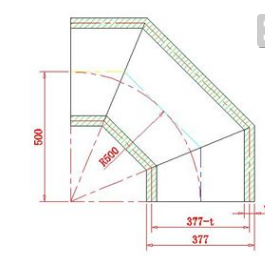24小时*7天为您服务

QQ：3764304

# 90度弯头的各种计算方式

90度弯头的展开图就是一个标准的余弦曲线,所以放样公式就是Y=COSX。

elbow在管路系统中，弯头是改变管路方向的管件。按角度分，有45°及90°180°三种最常用的，另外根据工程需要还包括60°等其他非正常角度弯头。弯头的材料有铸铁、不锈钢、合金钢、可锻铸铁、碳钢、有色金属及塑料等。90度弯头放样的计算方法。
直径300MM,弯度90度，这样下料：划一根直线长为∏(d-td---圆管外径，t---板厚)
把该线段16等分，从0.1.2.3......16。两节等径直角弯头放样计算计算式：Yn=r cosα当0°≤αn≤90°时 Yn=1/2(d-2t)cosα
当90°<αn≤180°时 Yn=1/2dcosα
式中Yn---展开图圆周长度等分点至曲线坐标值;
r---辅助圆半径;
d---圆管外径;
t---板厚;
αn---辅助圆周等分角度;直径为300mm的管，
n可选16α1=360°/16=22.5°α2=45°α3=67.5°α4=90°α5=112.5°α6=135°.α7=157.5°α8=180°
计算式：Y0=1/2(d-2t)cos0°=0.5(d-2t)
Y1=1/2(d-2t)cos22.5°=0.4619(d-2t)
Y2=1/2(d-2t)cos45°=0.3536(d-2t)
Y3=1/2(d-2t)cos67.5°=0.1913(d-2t)
Y4=1/2(d-2t)cos90°=0
Y5=1/2 dcos112.5°=-0.1913d
Y6=1/2 dcos135°=-0.3536d
Y7=1/2 dcos157.5°=-0.4619d
Y8=1/2 dcos180°=-0.5d
出现二个曲线，然而把这九个点连接起来，这是一半，另一半与其相同，这样做出的样板是90度弯头的一半。

QQ：3764304